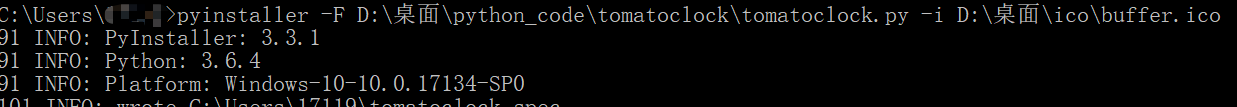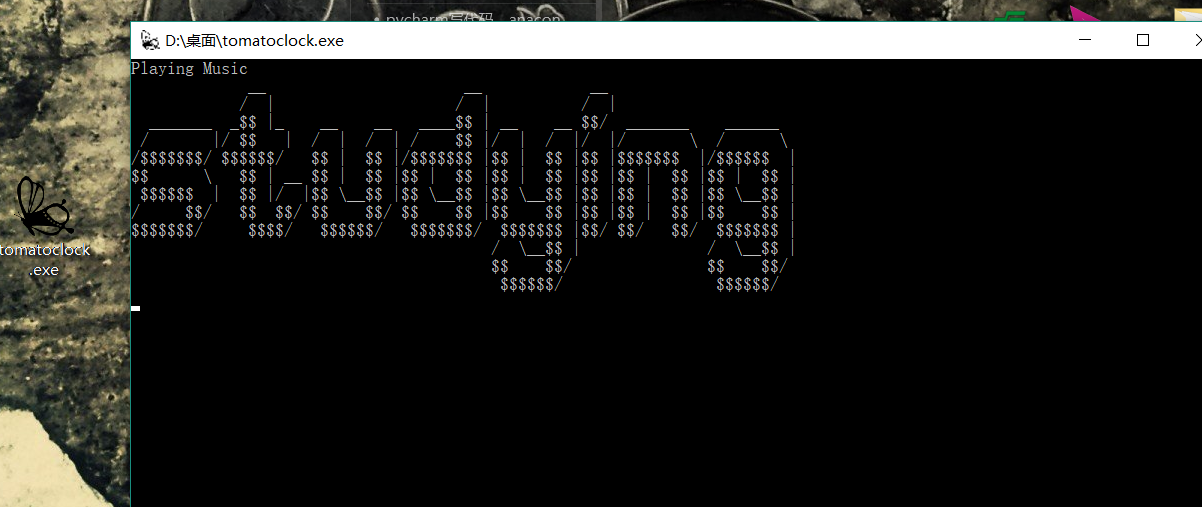# Python 做一个“番茄工作法” 的 小闹钟程序，提高学习效率啊 有没有？ 哈哈

The Pomodoro Technique is a time management method developed by Francesco Cirillo in the late 1980s. The technique uses a timer to break down work into intervals, traditionally 25 minutes in length, separated by short breaks. Each interval is known as a pomodoro...

import subprocess
music = r"D:\CloudMusic\represent.mp3"
subprocess.Popen(["start",music],shell=True)

while(true) : 学习： timesleep(学习时间） 休息 timesleep(休息时间）

import subprocess
import time

def playMusic(case):
if case == 1:
music = r"D:\CloudMusic\represent.mp3"
else:
music = r"D:\CloudMusic\kongbaige.mp3"
print("Playing Music")
subprocess.Popen(["start",music],shell=True)

def study():
print('''             __                      __            __
/  |                    /  |          /  |
_______  _$$|_ __ __ ____$$ | __    __ $$/ _______ ______ / |/$$   |  /  |  /  | /    $$|/ | / |/ |/ \ / \ /$$$/ $$/$$ | $$|/$$$ |$$|$$ |$$|$$$|/$$|$$ \ $$| __$$ | $$|$$ | $$|$$ | $$|$$ |$$|$$ |$$|$$ | $$|$$ |/ |$$\__$$ |$$\__$$ |$$\__$$ |$$|$$ | $$|$$ \__$$| /$$/ / /  | |$$|$$ | $$|$$ $$|$$$/     /   $$/$$$/ $$|$$/ $$/$$/ $$| / \__$$ | / \__$$|$$ $$/$$ $$/$$/ $$/ ''') def rest(): print(''' __ / | ______ ______ _______ _$$ |_ / \ / \ / |/ $$| /$$ |/$$|/$$$/ $$/$$ |  $$/$$    $$|$$      \   $$| __$$ |      /  $$|$$ |/  |
$$|$$       |/     $$/$$  $$/$$/        $$/$$\$/     /  ''')

#按分钟计时
def run(studyTime,interval):
while True:
try:
playMusic(1) #这里暂停就只能在网易云音乐里关掉了
study();
time.sleep(studyTime*60) #就是睡指定时间
print("学习暂停,进入5分钟休息时间")
playMusic(2)
rest()
time.sleep(interval*60)#回到循环开头
except Exception as e:
print(e)

if __name__ == "__main__":
studyTime = 25
interval = 5
run(studyTime,interval)


~/dist/xxxx.exe 里面   ~指你个人用户目录，windows一般 c://Users//xxxx/dist©️2019 CSDN 皮肤主题: 大白 设计师: CSDN官方博客